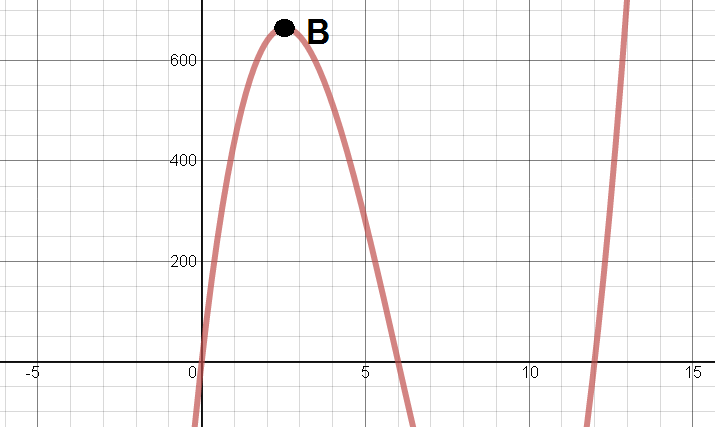# Maximizing Volume - Revisited

2 teachers like this lesson
Print Lesson

## Objective

SWBAT use derivatives to solve optimizations problems.

#### Big Idea

We are going to maximize volume again - but this time with derivatives.

## Launch

10 minutes

Way back in the first unit of the year we looked at a problem where students were to find the maximum volume assembled from a piece of paper. Today we are revisiting that problem but looking at it through a different lens - we are going to solve it using derivatives. I start today's lesson's by giving students this worksheet and have them work with their table groups for about 5 minutes to see if they remember how we solved this problem the first time.

We will discuss what students recall about this problem. Here are some things that students usually remember:

• We created the equation V = (24 - 2x)(24 - 4x)(x) where x represents the width of one fold.
• We graphed the equation and found the relative maximum since that represents the greatest possible volume.
• We used a graphing calculator to find the exact x-value. We plugged it back into the equation to find the maximum volume.

## Explore

20 minutes

Now that students recall how we solved this problem the first time, it is time to think about how we can use derivatives to solve. I will sketch the graph of the volume function and mark point B (shown below) as the relative maximum that represent the greatest possible volume.I will ask students how we can use derivatives to find this point instead of the graphing calculator. If no one can figure it out I will ask a student to sketch the tangent line at point B  and ask what we know about its slope. Since its slope is zero, the derivative must also be equal to zero, so we can set the derivative equal to zero to find that point. We will go through the process to demonstrate.

It is a good idea to talk about how there are two different points where the derivative is equal to zero. I will ask students how we know that the other point is not the maximum volume to get a few different strategies for figuring it out.

Next, I give students about 10 minutes to work on #2 on the worksheet. This is a new context so I monitor to make sure that students are setting up a function like we did for the volume problem.

## Share and Summarize

20 minutes

While students are working, I will look for a student who correctly set up the strength equation (for question #2 on this worksheet) using one variable to share their work. I will call on other students to interpret the work and explain how the student came up with these equations.

The biggest hurdle for this problem is using figuring out that we must use Pythagorean Theorem to set up an equation relating w and h in order to plug that into the strength equation. I always ask students why we can't just take the derivative of the strength equation to emphasize that we need to have a function with only one input in order to take the derivative.

After this problem I will recap our process to close the lesson. I discuss this recap in the video below.

Finally I give students this homework assignment to give them some more experience with these optimization problems.